Courses

# Viscous Flow of incompressible Fluid Civil Engineering (CE) Notes | EduRev

## Civil Engineering (CE) : Viscous Flow of incompressible Fluid Civil Engineering (CE) Notes | EduRev

The document Viscous Flow of incompressible Fluid Civil Engineering (CE) Notes | EduRev is a part of the Civil Engineering (CE) Course Topic wise GATE Past Year Papers for Civil Engineering.
All you need of Civil Engineering (CE) at this link: Civil Engineering (CE)

Question 1. A solid sphere of radius, r and made of material with density, ps is moving through the atmosphere (constant pressure, p) with a velocity, v. The net force ONLY due to atmospheric pressure (Fp) acting on the sphere at any time t, is    [2019 : 1 Mark, Set-ll]
(a) 4πr2p
(b)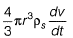(c)  πr2p
(d) zero

Answer: (d)

Question 2. Consider a laminar flow in the x-direction between two infinite parallel plates (Couette flow). The lower plate is stationary and the upper plate is moving with a velocity of 1 cm/s in the x-direction. The distance between the plates is 5 mm and the dynamic viscosity of the fluid is 0,01 N-s/m2. If the shear stress on the lower plate is zero, the pressure gradient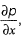(in N/m2 per m, round off to 1 decimal place) is  ________.     [2019 : 2 Marks, Set-I]
Solution: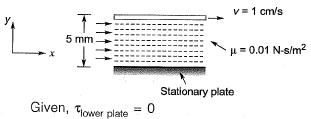Velocity distribution equation for Couette flow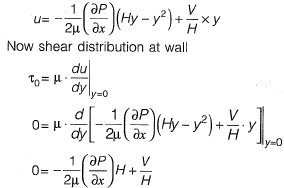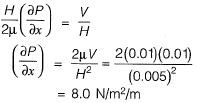Question 3.  For a steady incompressible laminar flow between two infinite parallel stationary plates, the shear stress variation is    [2017 : 1 Mark, Set-I]
(a) linear with zero value at the plates
(b) linear with zero value at the center
(c) quadratic with zero value at the plates
(d) quadratic with zero value at the center
Answer: (b)
Solution:
Shear stress variation between parallel stationary plats.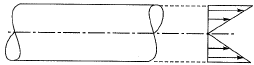Question 4. With reference to a standard Cartesian (x, y) plane, the parabolic velocity distribution profile of fully developed laminar flow in x-direction between two parallel, stationary and identical piates that are separated by distance, h, is given by the expression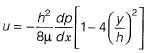In this equation, the y = 0 axis lies equidistant between the plates at a distance h/2 from the two plates, p is the pressure variable and n is the dynamic viscosity term. The maximum and average velocities are, respectively    [2014 : 2 Marks, Set-II]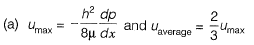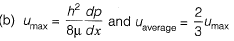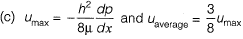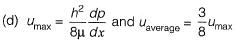Answer:
(a)
Solution: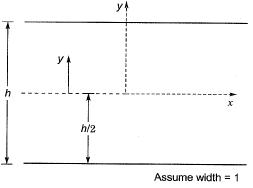Velocity expression for a laminar flow between two parallel plates is,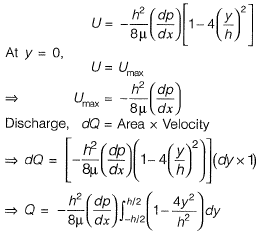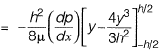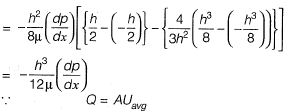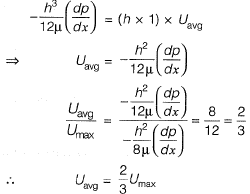Offer running on EduRev: Apply code STAYHOME200 to get INR 200 off on our premium plan EduRev Infinity!

69 docs

,

,

,

,

,

,

,

,

,

,

,

,

,

,

,

,

,

,

,

,

,

;